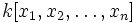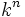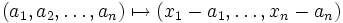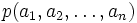# Max-spectrum of multivariate polynomial ring over an algebraically closed field

Let$k$ be an algebraically closed field. The max-spectrum of$k[x_1,x_2,\ldots,x_n]$ is described below.

## As a set

Further information: weak Nullstellensatz for algebraically closed fields

As a set, the max-spectrum is identified with$k^n$, where the identification is as follows:$(a_1,a_2,\ldots,a_n) \mapsto (x_1-a_1,\ldots, x_n-a_n)$

The left side denotes a point in$k^n$ and the right side denotes the maximal ideal for that point. Equivalently, we can think of the maximal ideal as the kernel of the "evaluation map" which sends a polynomial$p$ to the value$p(a_1,a_2,\ldots,a_n)$.

The above is one of the many formulations of the weak Nullstellensatz for algebraically closed fields.

## As a topological space

The topology that we give to the max-spectrum coincides with the usual Zariski topology we put on$k^n$.